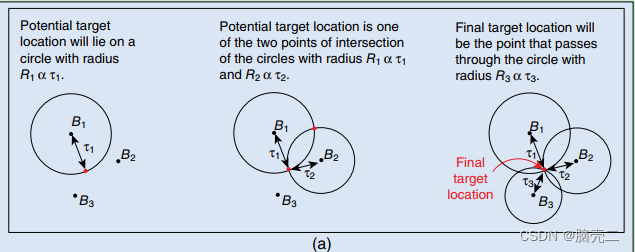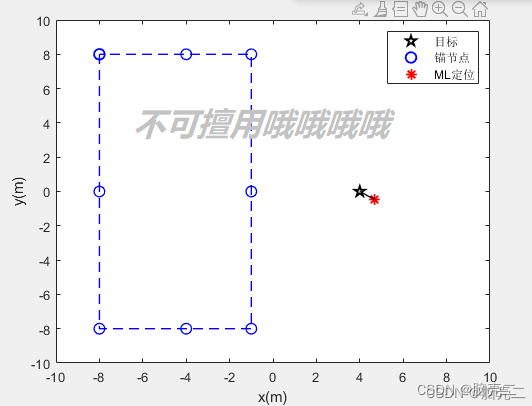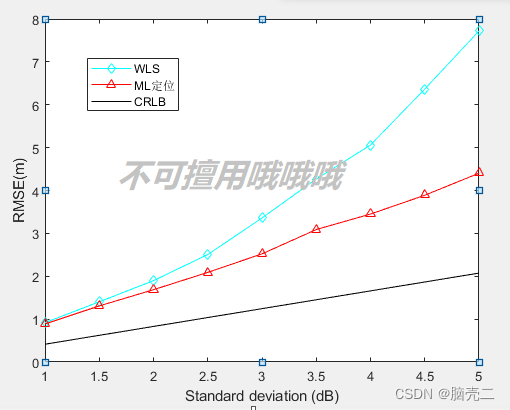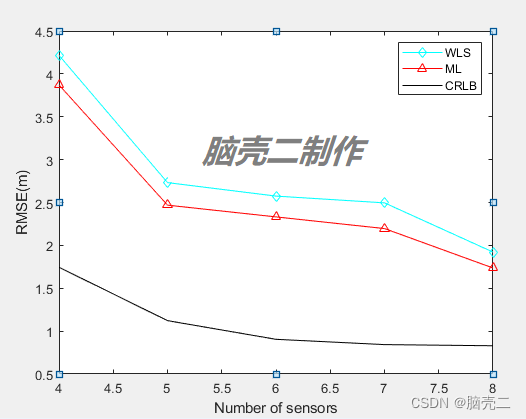WX: ZB823618313

• 1. 室内定位/无线传感器网络定位技术
• 1.1. 室内定位/无线传感器网络定位分类
• 1.2. 室内定位/无线传感器网络定位特点
• 1.3. 室内定位/无线传感器网络定位技术分类
• 1.4. 室内定位/无线传感器网络定位技术比较
• 4.1. 问题描述
• 4.2. ML估计
• 5. 仿真结果 1
• 5.1. 仿真场景（目标在传感器凸包外）
• 5.2. 定位误差RMSE(随着扰动增大)
• 5.3. 定位误差RMSE(随着传感器节点增多)

## 1. 室内定位/无线传感器网络定位技术

### 1.3. 室内定位/无线传感器网络定位技术分类

• 到达角度技术(AOA)
• 达到时间技术(TOA)
• 到达时间差技术(TDOA)

### 1.4. 室内定位/无线传感器网络定位技术比较

AOA 中、高 LOS 角度 只需要两个接收器。 不需要同步时间 需要天线阵列、价格昂贵
TOA LOS 距离 精度高 需要三个接收器、发射源和目标均时间同步
TDOA LOS 距离差 精度高，仅需要同步发射源的始终 LOS假设RSS测量模型制定如下。假设源发射功率为 P t P_t ，在没有干扰的情况下，第 i i 个传感器接收到的平均功率为 P i P_i ，模型为

Pi =Ki Pt di − α =Ki P0 ∣ ∣ x −xi ∣∣ − α ,   i = 1 , 2 , ⋯   , N P_i=K_iP_td_i^{-\alpha}=K_iP_0||\mathbf{x}-\mathbf{x}_i||^{-\alpha},~i=1,2,\cdots,N

r R S S , i =P0 − 10 α log ⁡ d id 0 +ni ,   i = 1 , 2 , ⋯   , N r_{RSS,i}=P_0 - 10\alpha\log\frac{d_i}{d_0}+n_i,~i=1,2,\cdots,N

x = [ x , y ] ′ \mathbf{x}=[x,y]' 为发射源的位置，即被定位的参数， y i = [ x i , y i ] ′ \mathbf{y}_i=[x_i,y_i]' 为第i个锚节点的位置，假设存在N个锚节点构成传感器网络，则 i = 1 , 2 , ⋯  , N i=1,2,\cdots,N n i n_i 为测量噪声，来源于RSS的干扰。

1-参数估计算法主要解决非移动目标（如传感器节点、发射源等）的定位问题，主要包括：
ML最大似然估计
LS最小二乘估计
WLS加权最小二乘估计
NLS非线性最小二乘估计

**注意：**这类方法也可以解决移动目标的室内外定位问题。

%%%%%%%%%%%%%%%%%%%%%%%%%%%%%%%%%%%%%%%%%%%%%%%%%

%%%%%%%%%%%%%%%%%%%%%%%%%%%%%%%%%%%%%%%%%%%%%%%%%

2- 滤波方法主要解决移动目标（如智能小车、移动机器人、移动终端等）的室内外定位问题，主要包括：

Divided difference filter DDF

… …

### 4.1. 问题描述

r R S S , i =P0 − 10 α log ⁡ d id 0 +ni ,   i = 1 , 2 , ⋯   , N r_{RSS,i}=P_0 - 10\alpha\log\frac{d_i}{d_0}+n_i,~i=1,2,\cdots,N

x = [ x , y ] ′ \mathbf{x}=[x,y]' 为发射源的位置，即被定位的参数， y i = [ x i , y i ] ′ \mathbf{y}_i=[x_i,y_i]' 为第i个锚节点的位置，假设存在N个锚节点构成传感器网络，则 i = 1 , 2 , ⋯  , N i=1,2,\cdots,N n i n_i 为测量噪声，来源于RSS的干扰。

### 4.2. ML估计

P (r R S S ∣ x ) =∏ i = 1 N 1 2 π σ i e −( r R S S , i − h ( x ) ) 2 2 σ i 2 P(\mathbf{r_{RSS}}|\mathbf{x})=\prod_{i=1}^N\frac{1}{\sqrt{2\pi}\sigma_i}e^{-\frac{(r_{RSS,i}-h(\mathbf{x}))^2}{2\sigma_i^2}}

h ( x ) =P0 − 10 α log ⁡ ∣ ∣ x − x i∣ ∣d 0h(\mathbf{x})=P_0 - 10\alpha\log\frac{||\mathbf{x}-\mathbf{x}_i||}{d_0}

L ( x ) = c +∑ i = 1 N −1 2 σ i 2 (r R S S , i − h ( x ))2 L(\mathbf{x})=c+ \sum_{i=1}^N-\frac{1}{2\sigma_i^2}(r_{RSS,i}-h(\mathbf{x}))^2

x ^ = arg ⁡ max ⁡ L ( x ) = arg ⁡ min ⁡ ∑ i = 1 N 1 2 σ i 2 ( r R S S , i − h ( x ) ) 2 \begin{aligned} \hat{\mathbf{x}}&=\arg \max L(\mathbf{x})\\ &=\arg \min \sum_{i=1}^N\frac{1}{2\sigma_i^2}(r_{RSS,i}-h(\mathbf{x}))^2 \end{aligned}

## 5. 仿真结果 1

### 5.1. 仿真场景（目标在传感器凸包外）TDOA定位结果:4.6807-0.4673

### 5.2. 定位误差RMSE(随着扰动增大)Target_position_ML =    4.6807    3.3259    3.3647    4.5194    2.8333    2.4684    8.5720    2.5546    7.5276   -0.4673    0.2277   -0.8136   -0.3219    1.1827    0.7298   -0.5827    0.3165    1.4475

### 5.3. 定位误差RMSE(随着传感器节点增多)Target_position_ML =   0  0   17.8782    3.8167    1.8567    8.8670    1.2396    1.7261   0  0    3.4926   -2.1692   -0.4536   -0.5194   -0.6874   -0.1439